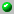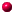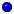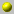Dennis Stanton

The homepage can be accessed here.
School of Mathematics
University of Minnesota
Minneapolis, Minnesota 55455
stanton@math.umn.edu

the following papers are available on our server= dvi format= ps format= tex format= pdf formata_convolution_formula_for_the_tutte_polynomiala_hypergeometric_hierarchy_for_the_andrews_evaluationscombinatorial_laplacians_of_matroid_complexesdeterminants_in_plane_partitions_enumerationsfake_gaussian_sequenceslattice_paths_and_positive_trigonometric_sumsmore_orthogonal_polynomials_as_momentsopen_positivity_conjectures_for_integer_partitionsquadratic_q_exponentials_and_connection_coefficient_problemsrim_hook_latticessieved_partition_functions_and_q_binomial_coefficientsthree_statistics_on_lattice_pathsthree_statistics_on_lattice_pathsunimodality_of_differences_of_specialized_schur_functions

Go back to the directory of pre and reprints.
Or search the database by keywords
Or look for the newest entires in the database